The proper way to dynamically create a two-dimensional array in C

When I began working with matrices in C, I was obsessed with malloc. I almost never defined static arrays, because I thought that malloc turned me into a ‘Memory God’ capable of managing memory efficiently and improving loading and response times of my application, all while not putting the burden on the operating system. So, I quickly figured out how to dynamically allocate a two dimensional array. This is what I used to do in order to create a 10×5 array of integers:

After the job was done, I used to free the matrix like this:

Someone might ask: “What’s wrong with that?”. Well, for most intents and purposes this code works perfectly fine, but there is one subtle issue with this code and chances are that, if you use something like that, you are not even aware of it. The problem with this code is that it goes against the way C allocates static matrices in memory.

When you create a static 3×3 matrix in C like this:

```int staticMatrix;
```

the memory blocks allocated for each column stand each next to each other as demonstrated below: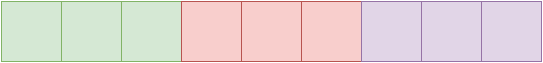The green blocks are the columns of the first row, the red blocks are the columns of the second row and the purple blocks are the columns of the third row. This two-dimensional array or array of arrays is actually a contiguous block in memory.

This is also the reason why the second dimension is always required when passing a 2D array to a function˙ the compiler needs to now the offset between rows, because in memory there are no actual dimensions. To better explain this, let’s assume you have this function that takes a 2D array and does some operations on it (we do not care about the exact operations):

```void workWithMatrix(int matrix[]);
```

We are required to specify the number of columns, but not the number of rows (although we can include that too, if we want). That’s because, every time we use the indexer operator, the compiler translates our statement into a pointer. If the following statement exists within workWithMatrix:

```int value = matrix;
```

The compiler will “translate it” to this:

```int value = *(matrix + 2*3 + 1);  //*(matrix + 7)
[\c]

If the statement was:

int value = matrix;
[\c]

It would translate into this:

int value = *(matrix + 1*3 + 1);  //*(matrix + 4)
```

Notice the number 3, that’s the offset, a.k.a. the number of columns, a.k.a. the number of blocks between two rows.

This is a visual illustration of how the compiler treats your 2D array as a single dimension one.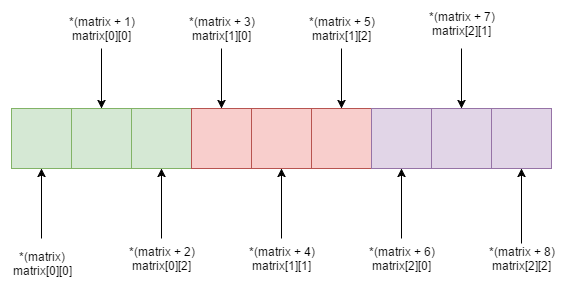The following is a typical illustration of how the dynamic matrix we created with malloc gets stored in memory: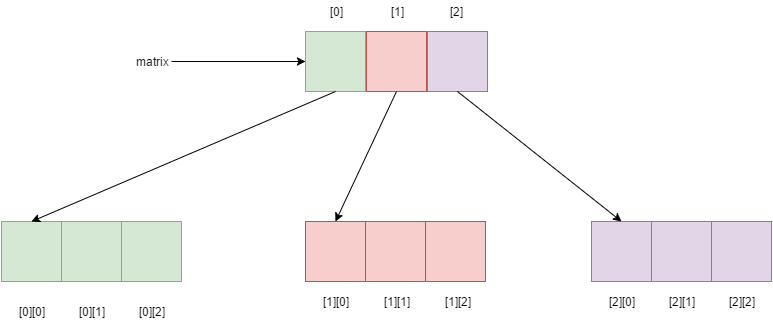As you can see, the blocks may be consecutive or they may not, there is no guarantee. That rarely causes a problem in most C programs, but when you have some advance logic, you want the matrix to be stored in contiguous blocks (for example, when creating a derived data type in MPI). To fix this, let’s introduce the correct way to dynamically allocate two dimensional arrays. What we want is to emulate a static array and from what we’ve seen, a statically defined 2D array is actually a one dimensional array (since there’s no such thing as a dimension in memory). So, when we want an NxM matrix, we first need to create a one dimensional array containing NxM elements, and then we create an array of pointers and make them point to the correct place in memory.

In the following example, N=3 and M=3.

In steps:

1. Create a one-dimensional array of 3×3=9 elements: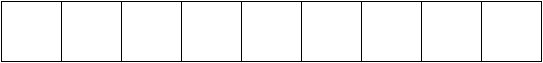2. Create a one-dimensional array of N=3 pointers (equal to the number of rows):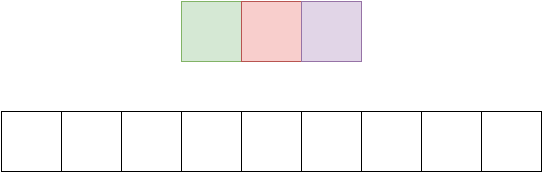3. Loop through the array of pointers and make them point to the start of each row, first one to array, second one to array and the third one to array: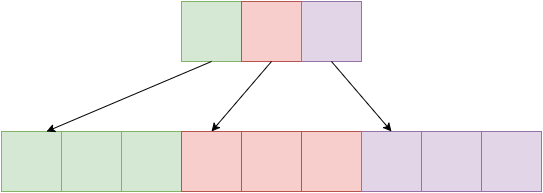4. Use the array of pointers (which is an int** for integers, a float** for floats, etc.) to reference the elements using the indexer operator []

And the long awaited code to achieve the above:

After we’re done with the matrix, we need to free any memory associated with it. To do that, we need to use a different approach than just looping through the rows and freeing the columns. This approach is actually faster and simpler. Straight to the code:

Remember that matrix is an array in and of itself. So we just need to free that, and also free the array of pointers. Pretty simple stuff.

I’ve also included some more helper functions for matrix operations. You can check them out here

I hope you liked this post and found it helpful. Have a nice day!